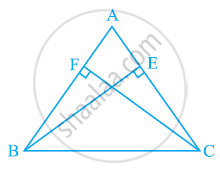# ABC is an isosceles triangle in which altitudes BE and CF are drawn to equal sides AC and AB respectively (see the given figure). Show that these altitudes are equal. - Mathematics

ABC is an isosceles triangle in which altitudes BE and CF are drawn to equal sides AC and AB respectively (see the given figure). Show that these altitudes are equal.#### Solution

In ΔAEB and ΔAFC,

∠AEB and ∠AFC (Each 90º)

∠A = ∠A (Common angle)

AB = AC (Given)

∴ ΔAEB ≅ ΔAFC (By AAS congruence rule)

⇒ BE = CF (By CPCT)

Concept: Properties of a Triangle
Is there an error in this question or solution?

#### APPEARS IN

NCERT Class 9 Maths
Chapter 7 Triangles
Exercise 7.2 | Q 3 | Page 124